2020-02-13 09:47:52

## 这里有8个流行的Python可视化工具包，你喜欢哪个？

50 / 0 / 0 / 0

Matplotlib、Seaborn 和 Pandas

Matplotlib 是比较低级的库，但它所支持的自定义程度令人难以置信（所以不要简单地将其排除在演示所用的包之外！），但还有其它更适合做展示的工具。

Matplotlib 还可以选择样式（style selection），它模拟了像 ggplot2 和 xkcd 等很流行的美化工具。下面是我用 Matplotlib 及相关工具所做的示例图：

import seaborn as sns
import matplotlib.pyplot as plt

color_order = ['xkcd:cerulean', 'xkcd:ocean',
'xkcd:black','xkcd:royal purple',
'xkcd:royal purple', 'xkcd:navy blue',
'xkcd:powder blue', 'xkcd:light maroon',
'xkcd:lightish blue','xkcd:navy']

sns.barplot(x=top10.Team,
y=top10.Salary,
palette=color_order).set_title('Teams with Highest Median Salary')

plt.ticklabel_format(style='sci', axis='y', scilimits=(0,0))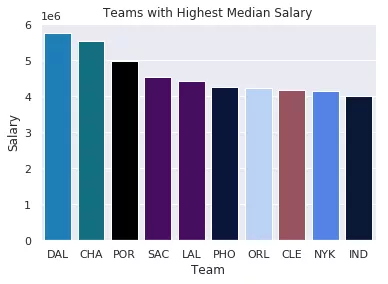import matplotlib.pyplot as plt
import scipy.stats as stats

#model2 is a regression model
log_resid = model2.predict(X_test)-y_test
stats.probplot(log_resid, dist="norm", plot=plt)
plt.title("Normal Q-Q plot")
plt.show()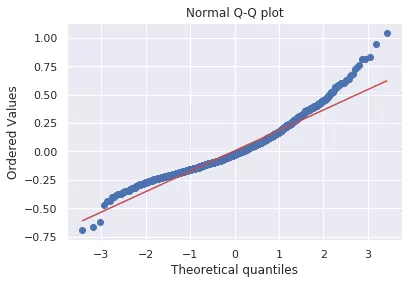ggplot(2)

ggplot2（我觉得也包括 Python 的 ggplot）举足轻重的原因是它们用「图形语法」来构建图片。基本前提是你可以实例化图，然后分别添加不同的特征；也就是说，你可以分别对标题、坐标轴、数据点以及趋势线等进行美化。

#All Salaries
ggplot(data=df, aes(x=season_start, y=salary, colour=team)) +
geom_point() +
theme(legend.position="none") +
labs(title = 'Salary Over Time', x='Year', y='Salary (\$)')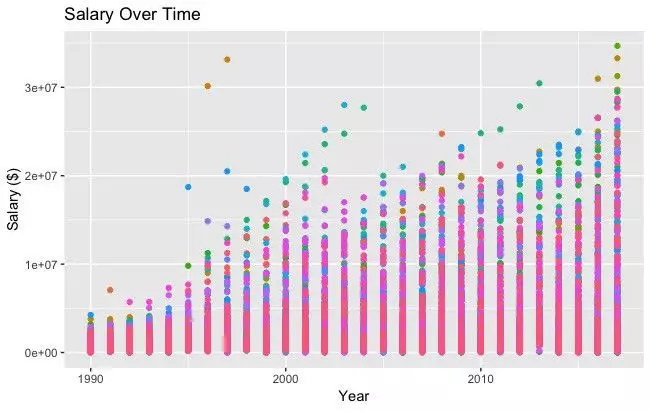Bokeh

Bokeh 很美。从概念上讲，Bokeh 类似于 ggplot，它们都是用图形语法来构建图片，但 Bokeh 具备可以做出专业图形和商业报表且便于使用的界面。为了说明这一点，我根据 538 Masculinity Survey 数据集写了制作直方图的代码：

import pandas as pd
from bokeh.plotting import figure
from bokeh.io import show

# is_masc is a one-hot encoded dataframe of responses to the question:
# "Do you identify as masculine?"

#Dataframe Prep
counts = is_masc.sum()
resps = is_masc.columns

#Bokeh
p2 = figure(title='Do You View Yourself As Masculine?',
x_axis_label='Response',
y_axis_label='Count',
x_range=list(resps))
p2.vbar(x=resps, top=counts, width=0.6, fill_color='red', line_color='black')
show(p2)

#Pandas
counts.plot(kind='bar')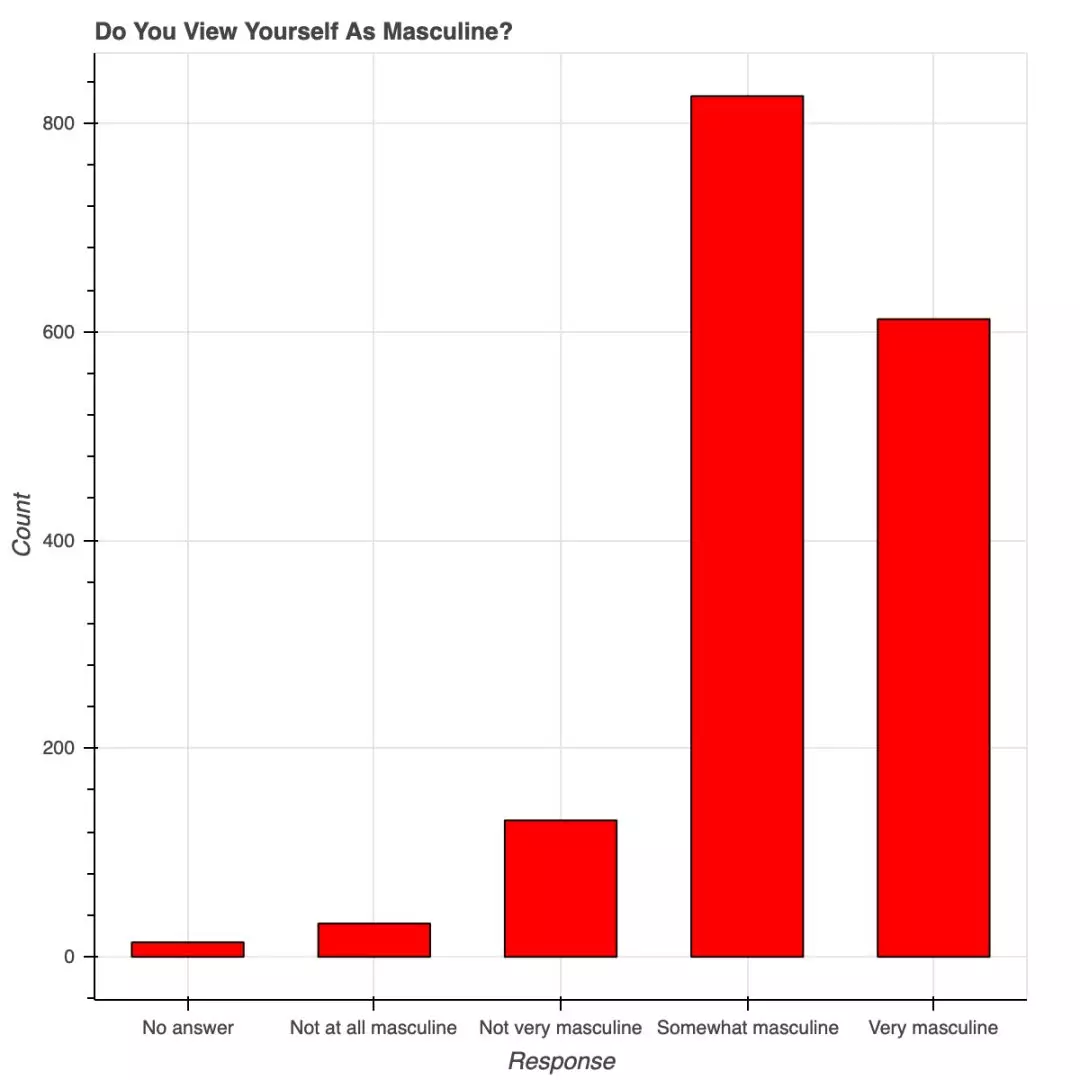<>用 Bokeh 表示调查结果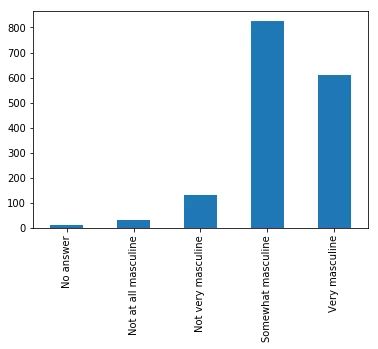<>用 Pandas 表示相同的数据

Bokeh 提供的所有便利都要在 matplotlib 中自定义，包括 x 轴标签的角度、背景线、y 轴刻度以及字体（大小、斜体、粗体）等。下图展示了一些随机趋势，其自定义程度更高：使用了图例和不同的颜色和线条。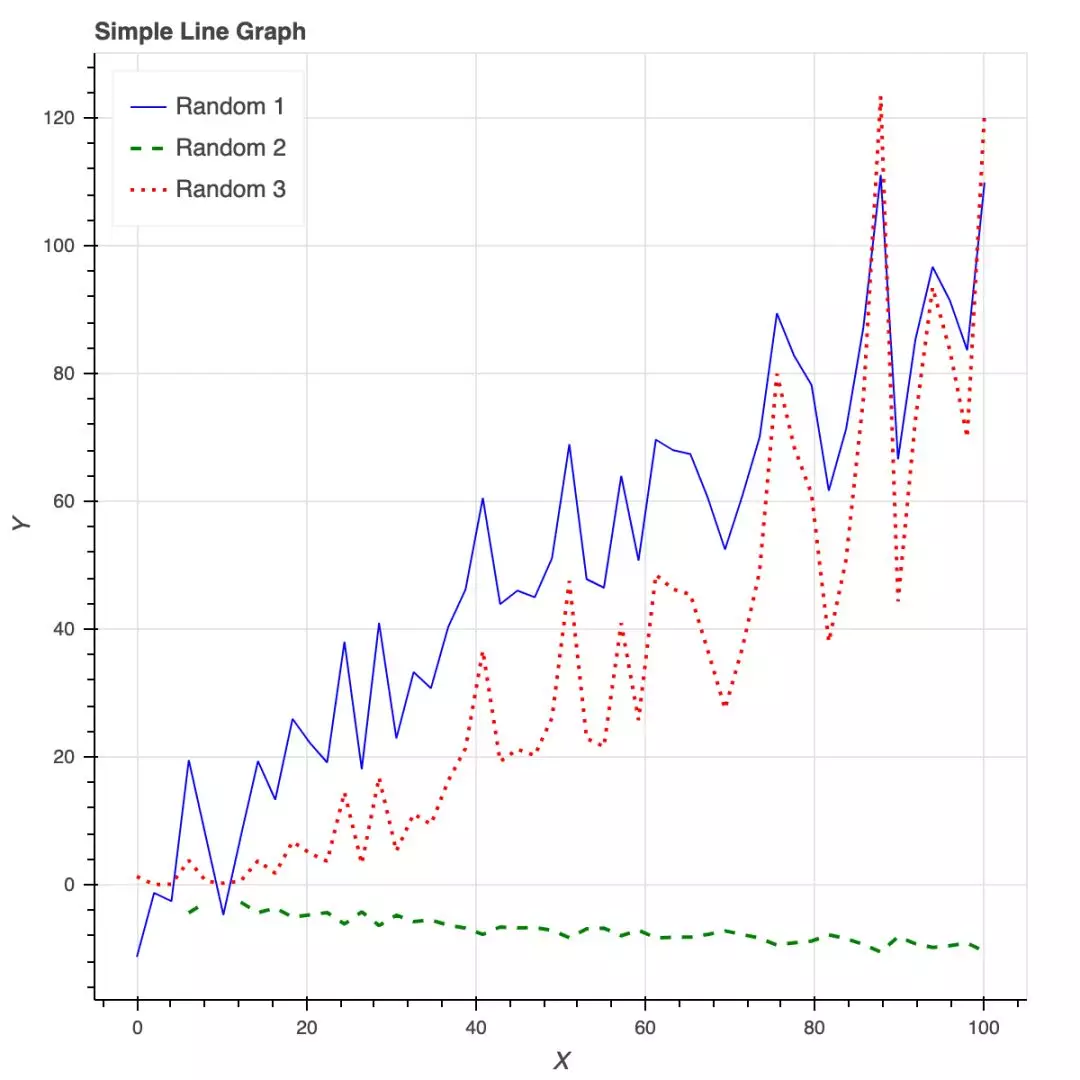Bokeh 还是制作交互式商业报表的绝佳工具。

Plotly

Plotly 非常强大，但用它设置和创建图形都要花费大量时间，而且都不直观。在用 Plotly 忙活了大半个上午后，我几乎什么都没做出来，干脆直接去吃饭了。我只创建了不带坐标标签的条形图，以及无法删掉线条的「散点图」。Ploty 入门时有一些要注意的点：

• 安装时要有 API 秘钥，还要注册，不是只用 pip 安装就可以；

• Plotly 所绘制的数据和布局对象是独一无二的，但并不直观；

• 图片布局对我来说没有用（40 行代码毫无意义！）

• 你可以在 Plotly 网站和 Python 环境中编辑图片；

• 支持交互式图片和商业报表；

• Plotly 与 Mapbox 合作，可以自定义地图；

• 很有潜力绘制优秀图形。

#plot 1 - barplot
# **note** - the layout lines do nothing and trip no errors
data = [go.Bar(x=team_ave_df.team,
y=team_ave_df.turnovers_per_mp)]

layout = go.Layout(

title=go.layout.Title(
text='Turnovers per Minute by Team',
xref='paper',
x=0
),

xaxis=go.layout.XAxis(
title = go.layout.xaxis.Title(
text='Team',
font=dict(
family='Courier New, monospace',
size=18,
color='#7f7f7f'
)
)
),

yaxis=go.layout.YAxis(
title = go.layout.yaxis.Title(
text='Average Turnovers/Minute',
font=dict(
family='Courier New, monospace',
size=18,
color='#7f7f7f'
)
)
),

autosize=True,
hovermode='closest')

py.iplot(figure_or_data=data, layout=layout, filename='jupyter-plot', sharing='public', fileopt='overwrite')

#plot 2 - attempt at a scatterplot
data = [go.Scatter(x=player_year.minutes_played,
y=player_year.salary,
marker=go.scatter.Marker(color='red',
size=3))]

layout = go.Layout(title="test",
xaxis=dict(title='why'),
yaxis=dict(title='plotly'))

py.iplot(figure_or_data=data, layout=layout, filename='jupyter-plot2', sharing='public')

[Image: image.png]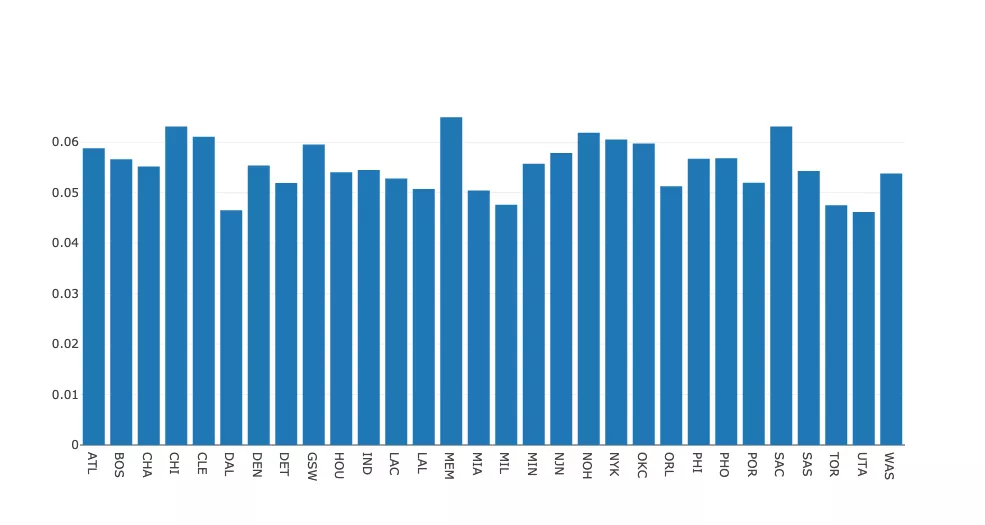<>表示不同 NBA 球队每分钟平均失误数的条形图。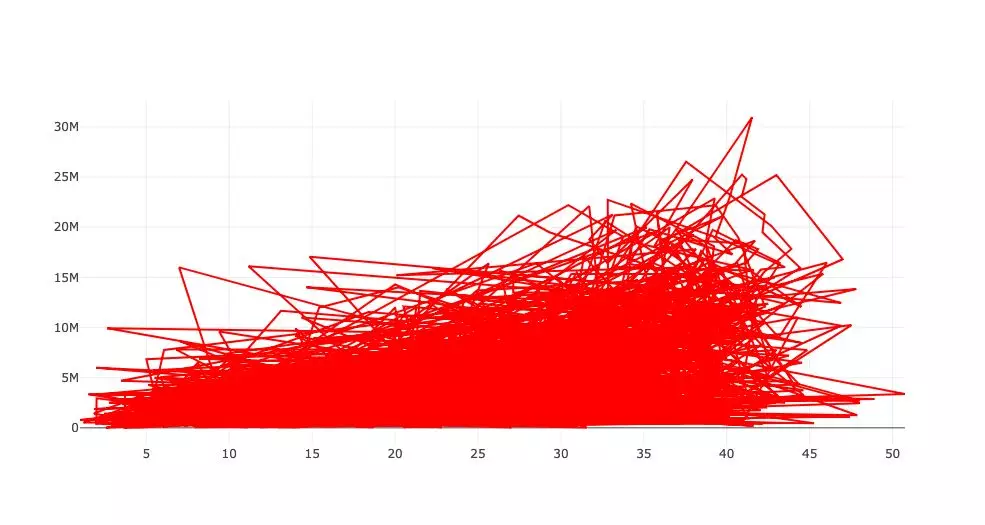<>表示薪水和在 NBA 的打球时间之间关系的散点图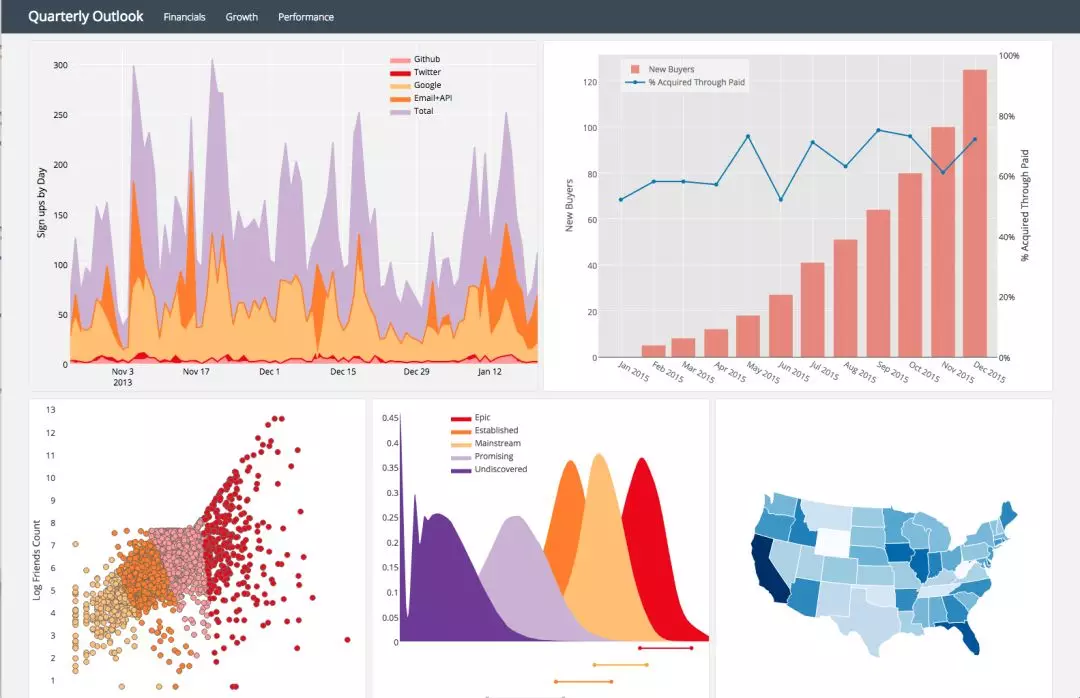<>Plotly 页面上的一些示例图Pygal**

Pygal 的名气就不那么大了，和其它常用的绘图包一样，它也是用图形框架语法来构建图像的。由于绘图目标比较简单，因此这是一个相对简单的绘图包。使用 Pygal 非常简单：

• 实例化图片；

• 用图片目标属性格式化；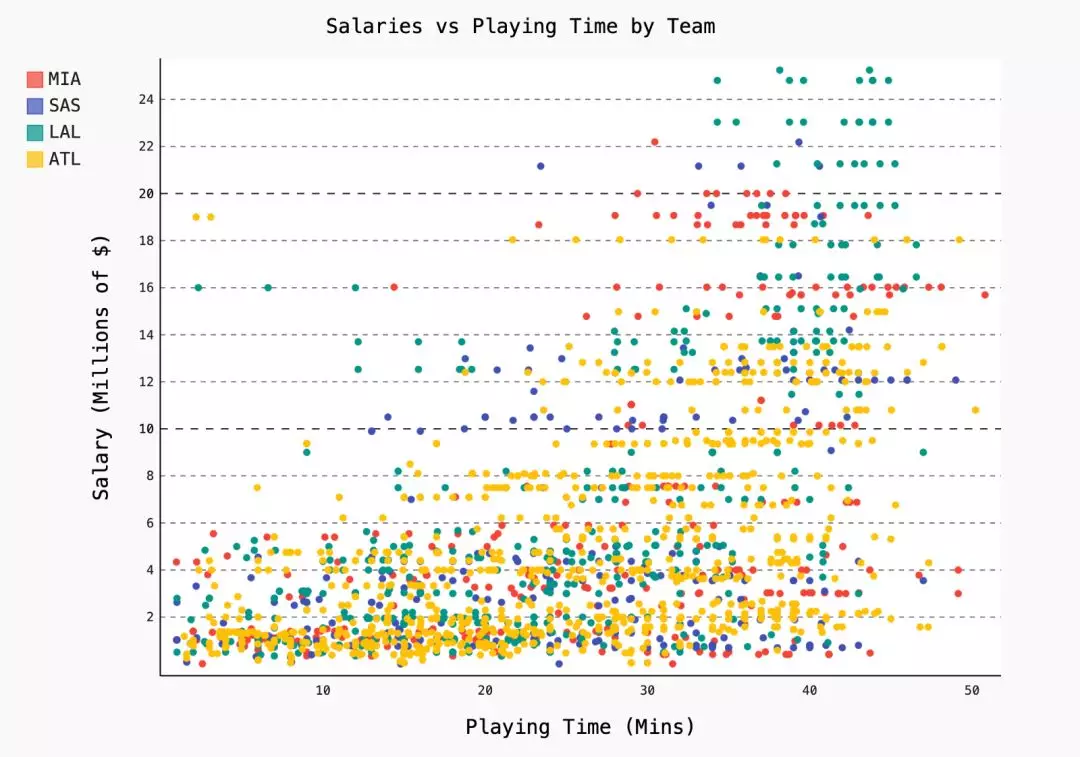Networkx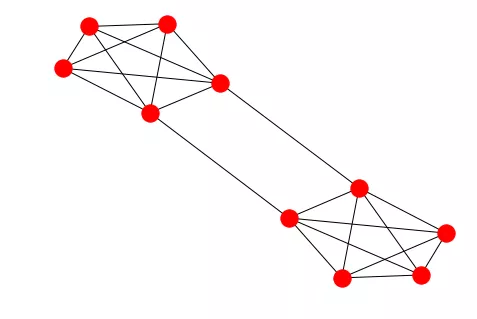options = {
'node_color' : range(len(G)),
'node_size' : 300,
'width' : 1,
'with_labels' : False,
'cmap' : plt.cm.coolwarm
}
nx.draw(G, **options)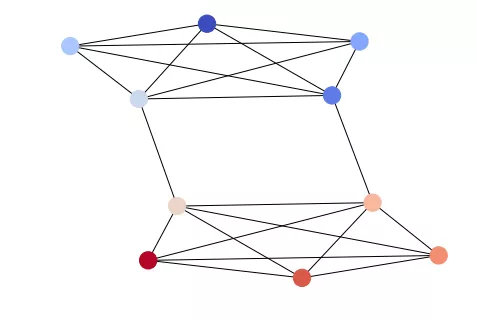import itertools
import networkx as nx
import matplotlib.pyplot as plt

circles = [line.split() for line in f]
f.close()

network = []
for circ in circles:
cleaned = [int(val) for val in circ[1:]]
network.append(cleaned)

G = nx.Graph()
for v in network:

edges = [itertools.combinations(net,2) for net in network]

for edge_group in edges:

options = {
'node_color' : 'lime',
'node_size' : 3,
'width' : 1,
'with_labels' : False,
}
nx.draw(G, **options)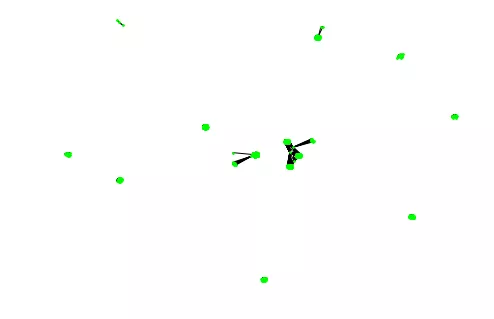PS: 如本文对您有疑惑，可加QQ：1752338621 进行讨论。

0
0